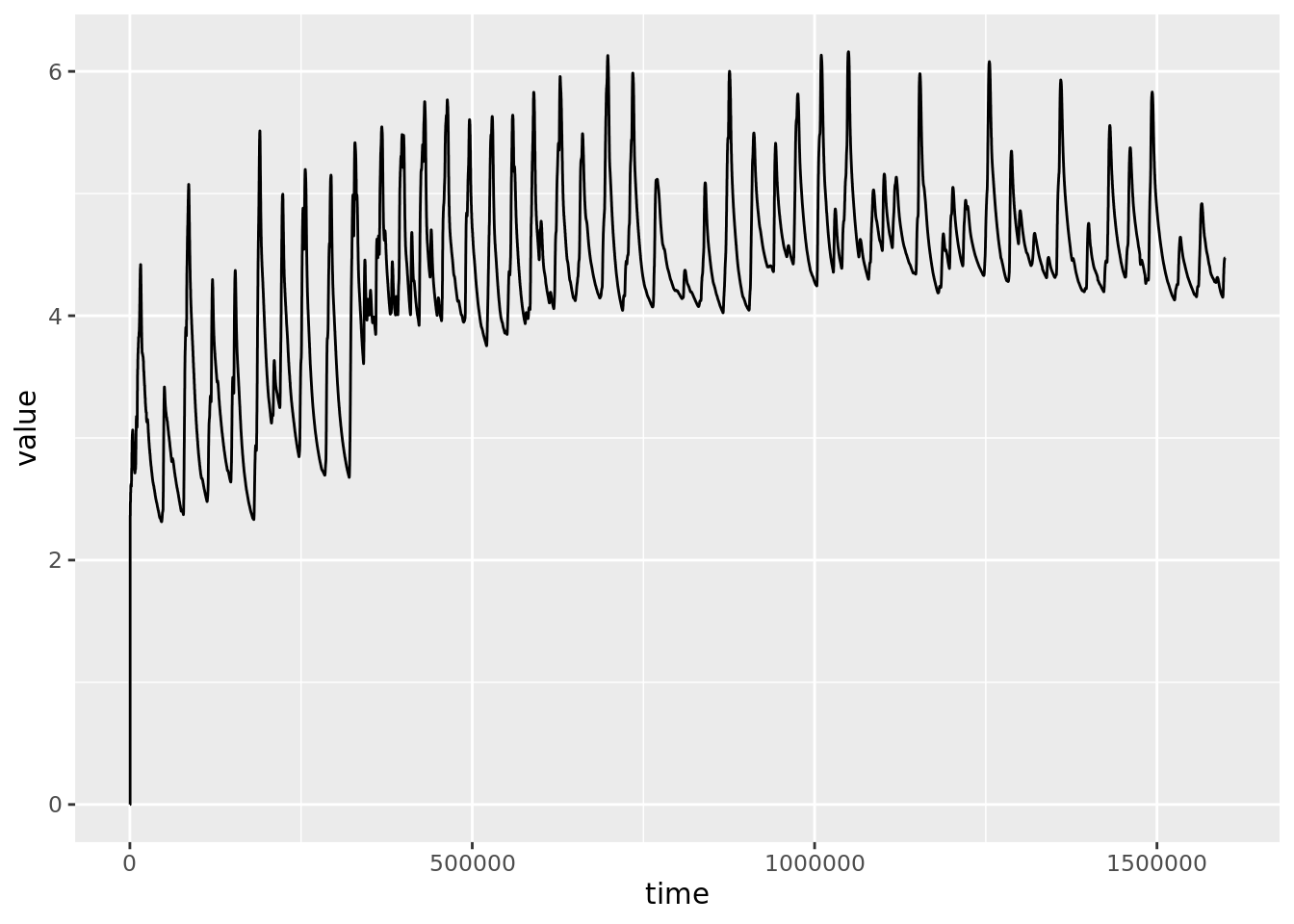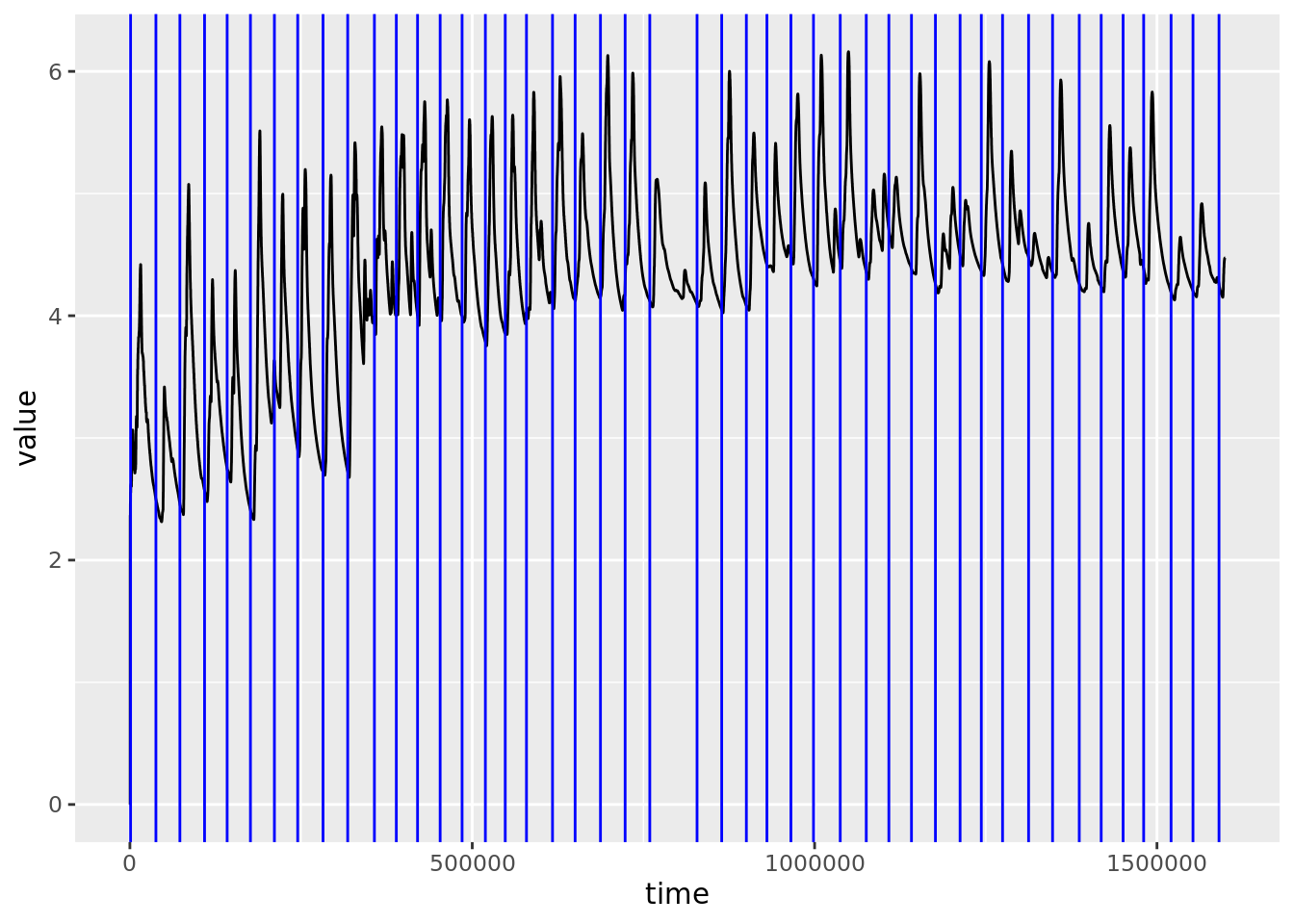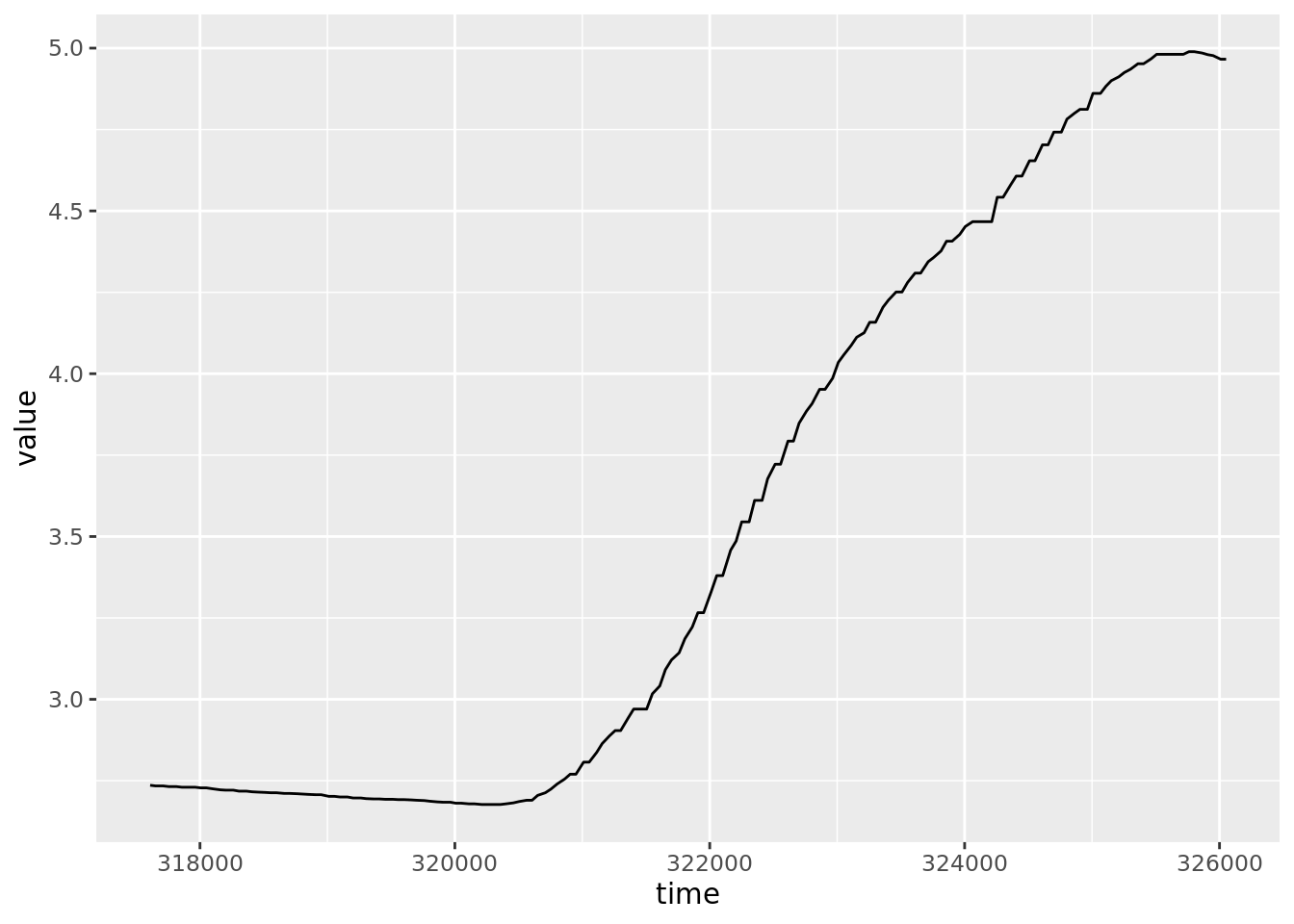## Before you start…

This is an advanced worksheet, which assumes you have completed the Absolute Beginners’ Guide to R course, the Research Methods in Practice (Quantitative section) course, and the Intermediate Guide to R course.

## Overview

This worksheet describes a full analysis pipeline for an undergraduate student dissertation on the Perruchet Effect. The experiment was basically identical to the experiment described by McAndrew et al. (2012), except that the electric shock was replaced by a loud unpleasant noise (a metal garden fork scraping across slate). For details of the procedure, see the McAndrew paper, but here’s a very brief summary:

This was a conditioning experiment. A shape (brown cylinder, known as a conditioned stimulus, or ‘CS’) appears on the screen for 8 seconds. On 50% of occasions, this is then followed by the unpleasant noise (the unconditioned stimulus or ‘US’). The participant’s reaction to each CS is measured in two ways:

1. While the CS is on the screen, they are asked to rate their expectancy of the US on each trial, using a numerical scale. Higher ratings indicate greater conscious expectation of the unpleasant noise.

2. Throughout the experiment, their Galvanic Skin Response, a.k.a. electrodermal activity is measured. This is a measure of physiological arousal, greater numbers mean more arousal. Higher arousal is interpreted as greater unconscious expectancy of the unpleasant noise.

So, we have two dependent variables - expectancy ratings, and GSR. There is also one independent variable - “run length”. This relates to how many trials in a row you have experienced the unpleasant noise. For example, a “+3” trial means you have experienced the noise three times in a row; a “-2” trial means you have experienced the absence of the noise two trials in a row. The run lengths in this experiment range from -3 to +3.

There are two predictions. The first prediction is that the GSR will increase with run length, because the unconscious association between the shape and the noise will grow with repeated pairings. The second prediction is that the conscious expectancies will do the opposite - they will decrease with run length. This is known as Gamblers’ Fallacy - in this case, the belief that, even though the sequence is random, a run of unpleasant noises means the next trial is more likely to be silent. This result is often taken to be one of the strongest pieces of evidence for a dual-process theory of learning, i.e. that we have unconscious and conscious learning processes that act somewhat independently of each other. This is because it can be hard to see how a single learning process could believe two things at the same time e.g. that on the +3 trials, it was simultaneously most and least likely that an unpleasant noise would occur.

## Getting data from a public repository

A basic principle of open science is that you publicly share your raw data (in anonymized form). Many psychologists, including the ones who ran this study, choose the OSF website to do this. So, the first thing you need to do is download the data from OSF. Of course, there’s an R package for that (`osfr`).

So, first, create a new project in R.

Next, create a new, empty R script and save it as `perruchet.R`. Put all the commands from this worksheet into `perruchet.R`, and run them from there. Save your script regularly.

Enter these commands into your script, and run them:

``````## Load data from OSF (commands only need to be run once)
library(osfr)
proj  <- osf_retrieve_node("r7axw")
files  <- osf_ls_files(proj)

### Explanation of commands

The first line is a comment. These are ignored by R, but make your script easier for humans to read. In this case, the comment reminds the human reader that you only need to download the data from OSF once.

The second line loads the `osfr` package.

The third line asks the OSF website for the information it needs to download the data. To do this, we need the last part of the web address. This repository is at https://osf.io/r7axw/, so we need to use `r7axw`.

The fourth line gets a list of the files in the repository, much as we did for local files in Preprocessing data from experiments.

The final file actually downloads those files to your R project. In this case, you’ll find that you now have a directory called `data`, which contains those files. The component `conflicts = "skip"` means that, if these files are already in your project, you should not overwrite them, but leave them as they are.

You can ignore the file `MAIN.csv`, which we’re not going to use. The first file you should open is `codebook.md`. A codebook is a file that explains what each of the columns in a data files means. Read the codebook and, while you’re reading through, look at the data file it is talking about, `DATA.csv`. It is this file on which all the following analysis is based.

As with any analysis, the first step is to load the data into R.

Enter these commands into your script, and run them:

``````## Load packages, data. Rename data frame columns
library(tidyverse)
colnames(raw) <- c("subj", "time", "key", "value")``````

### Explanation of commands

The second and third lines should be familiar from previous worksheets; we nearly always need the `tidyverse` package, and we use the `read_csv` command to load the data. The `data/` part of the filename `data/DATA.csv` says that the file `DATA.csv` is to be found in the directory (folder) called `data`.

The final line renames the columns, as covered in the Preprocessing data from experiments worksheet. I didn’t like the names of the columns very much so I changed them to something more comprehensible and quicker to type, using the `colnames` command.

## De-duplicating data

We’re now going to get the data into a state where we can meaningfully analyse it. The following commands were fully explained in the data preprocessing for experiments worksheet.

Enter these commands into your script, and run them:

``````## De-duplicate data; remove pilot study
raw <- distinct(raw)
raw  <- raw %>% filter(subj > 161)``````

## Excluding participants

Participants in this experiment were told to rate their expectancy of the US during the CS, on every trial. However, they only had 8 seconds to do this, and the experimenters noticed that sometimes people failed to make a rating. The experimental apparatus records this as a rating of zero.

Participants who miss a lot of ratings aren’t really following the instructions, making their data hard to interpret. In such cases, we normally exclude those participants from further analysis. The following commands were fully explained in the data preprocessing for experiments worksheet.

Enter these commands into your script, and run them:

``````## Number of expectancy ratings, by participant
inspect  <- raw %>% filter(key == "ER") %>% filter(value == 0)
table(inspect\$subj)``````
``````
163 164 166 176 184 188 191 196 199 202 223 233 235 236 239 246
1  19  46  16   2   3   2   1  32   3   3   1   3   9  34   1 ``````
``````## Exclude participants
exclude  <- c(164, 166, 176, 199, 236, 239)
raw  <- raw %>% filter(!(subj %in% exclude))``````

### Note

There are still a few trials where the participant makes no expectancy rating, and the computer records this as an expectancy of zero. There’s a good case to be made for recoding the rating on those trials as either `NA` (meaning ‘missing data’) or `3`, meaning “participant is unsure whether the noise will occur”. For brevity, we don’t make these changes here, but once you’ve gone through the whole worksheet, perhaps try to work out how one might do this.

## Graphing GSR

The GSR data from this experiment is very rich, with a skin conductance measure taken from each participant approximately twenty times a second throughout the whole 30-40 minutes of the experiment. To ‘get a feel’ for these data, and also to check that the equipment was working properly, we need to plot that GSR as a graph, with time on the x-axis and GSR on the y-axis. We’ll need to do this one participant at a time, otherwise the graph will be really hard to read.

Enter these commands into your script, and run them:

``````## GSR plot
dat  <- raw %>% filter(subj == 168)
gsr <- dat %>% filter(key == "GS")
gplot <- gsr %>% ggplot(aes(x=time, y=value)) + geom_line()
gplot``````### Explanation of commands

The second line should be familiar, we’ve filtered the data to include just one participant (168).

The third line is also a simple filter operation - we’re keeping just those rows where the `key` column says `GS`. From `codebook.md`, we can see that this means the rows of the data file that contain GSR readings.

The fourth line should also be pretty familiar, we’re just doing a standard line graph, as we previously covered in, for example, the Understanding Interactions worksheet. In this case, we save the line plot in an object called `gplot`, and then show the graph by typing `gplot` as the last command. We do it this way so we can add other things to the graph later.

### Explanation of output

We can see the GSR going up and down over time. Why is it doing that? Our assumption is that this is people reacting to the CS (cylinder), which is sometimes followed by the US (noise). We can check this by adding a vertical line to the graph for the time each CS occurs.

## Adding CS onsets to our graph

We can add CS onset times like this; enter these commands into your script, and run them:

``````## Add CS onset times to graph
cs <- dat %>% filter(key == "CO")
gplot <- gplot + geom_vline(data=cs, mapping=aes(xintercept=time),
colour="blue")
gplot``````### Explanation of commands

The second line should be familiar - we are selecting those rows of the data that contain the key `CO`, which `codebook.md` tells us are the timings of the CS onsets.

We’ve also come across `geom_vline` before, as part of the Putting R to work worksheet - it just plots a vertical line at a place we ask it to. The new part of this command is `data=cs, mapping=aes(xintercept=time)`. Previously, we’ve just told R manually where we want the line (e.g. `xintercept = 4.35`). Now, we’re telling it that we want a bunch of lines, that the `data` for the position of those lines is to be found in `cs` data frame, and that the `time` column of that data frame should be used to find them.

Here, we’re adding the vertical lines to the plot we’ve already made `gplot <- gplot + geom_vline...`, and then showing the updated graph, `gplot`.

### Explanation of output

There appears to be a missing blue line in the middle. Actually, there isn’t, it’s just that the participant was given a break half way through the experiment, so there wasn’t a CS for a while. What we can also see, however, is that the GSR seems to go up each time there’s a CS, and then go back down again. Sometimes that’s quite a big rise, and sometimes, less big. If the experiment worked out as we expected, then the size of the GSR reaction will depend on the run length. We’ll look at this later on, but first we need to do some preprocessing.

## Baseline correction

One problem with GSR data (and much other neuroscience data, e.g. EEG, fMRI) is that it’s both highly variable (“noisy”), and changes systematically over time (“drift”). By ‘noisy’, we mean that, for a given event in the world, the GSR response to that event varies quite a lot, even if the event itself is objectively identical. In order to deal with this noise, we average the participant’s GSR over several trials, and so get a sense of what their typical response is.

By ‘drift’, we mean that these differences across trials are not random, they are affected by systematic changes in the participant over time. For example, as they hear more and more unpleasant noises, perhaps the noise becomes less unpleasant to them (“habituation”), and so their GSR reaction late in the experiment might be smaller than their GSR reaction early in the experiment. Another example – once someone’s GSR goes up, it can take quite a lot of time to go back down again (“return to baseline”). In this experiment, we waited a long time between trials, the inter-trial interval was around 30 seconds. However, this is often not enough, and we find that sometimes their GSR has not returned to baseline by the time the next CS appears.

The combination of noise and drift means that, not only do we need to average across a lot of trials, we also need to try and make those trials more comparable to each other before we average them. The process we use to do that here is baseline correction. This is simply a subtraction. We calculate the average GSR response in the 500 ms before the CS arrives (the baseline), and then we subtract that baseline from all the GSR readings while the CS is present.

Let’s illustrate this with a single trial from participant 168. For no particular reason, let’s take trial 10.

Enter these commands into your script, and run them:

``````## Plot a single trial
onset  <- cs\$time
full <- gsr %>% filter(time >= onset - 500 & time <= onset + 8000)
full %>% ggplot(aes(x=time, y=value)) + geom_line()``````### Explanation of commands

Line 2 - In order to find a single trial, we need to know at what time the CS began on that trial. We already have those CS onset times from doing the last graph, so we just ask R for the `10`th row of the `time` column of that `cs` data frame - `cs\$time` - and record it as `onset` (with `onset <-`)

Line 3 - Now we know where the CS starts, we need to take all the GSR readings from 500 ms before that (our baseline), up to 8000 ms after that (when the CS ends). We use the now-familiar `filter` command to do this.

Line 4 - Just a plain old line graph.

### Explanation of output

What we see in the graph is just a ‘zoomed in’ part of the previous graph - for the 10th trial, 500 ms before the CS onset to 8000ms after the CS onset. The x-axis has large numbers because time is counted from the start of the experiment, in milliseconds. The line looks a bit ‘jagged’ because the equipment is has limited accuracy in measuring GSR.

## Applying baseline correction

Now, we need to do two things.

First, we need to apply the baseline correction to these data. In other words, we work out the average GSR in the 500ms before the CS arrives; we then subtract that average from every data point, so the first 500 ms of the graph will always be around zero.

Second, we’re going to need to average all these trials, so we need the x-axis to be the same for all of them. To do this, we subtract the onset time from all the values on the x-axis, meaning we end up with data that goes from -500 ms to +8000 ms.

We can do all this in four lines of code, plus one to plot the new graph.

Enter these commands into your script, and run them:

``````## Basline correct that trial, and replot
precs  <- gsr %>% filter(time >= onset - 500 & time < onset)
correct  <- mean(precs\$value)
full <- full %>% mutate(cval = value - correct)
full <- full %>% mutate(rtime = time - onset)
full %>% ggplot(aes(x=rtime, y=cval)) + geom_line()``````